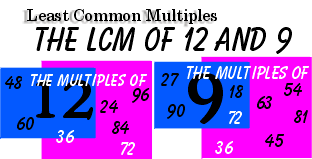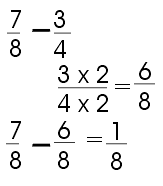In order to add or subtract fractions with unlike denominators, a common denominator is needed. We suggest the smallest common denominator be used. This common multiple is known as the Least Common Multiple (LCM). In the case of fractions, it is called the Least Common Denominator(LCD).In this example, both 36 and 72 are common multiples. The LCM is 36.Dalia and Vincent work in construction. Dalia gets every 4th day off in a row and Vincent gets every 5th day off in a row. They went out for the first time yesterday (when they both had the day off). How many more days will they have to wait till they can go out for the day again?In the above example, the fraction 3/4 must be converted to 8ths. 8 is the LCD for the two fractions.What was the total amount of Jack's fuel?HINT: Use / for fractions and leave a space between the whole number and the fraction.

Borrowing

Fractions